The OpenFOAM FoundationLLTMatrix< Type > Class Template Reference

Templated class to perform the Cholesky decomposition on a symmetric positive-definite matrix. More...

Inheritance diagram for LLTMatrix< Type >: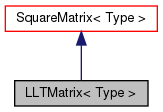[legend]
Collaboration diagram for LLTMatrix< Type >:[legend]

## Public Member Functions

LLTMatrix ()
Construct null. More...

LLTMatrix (const SquareMatrix< Type > &M)
Construct from a square matrix and perform the decomposition. More...

void decompose (const SquareMatrix< Type > &M)
Perform the Cholesky decomposition of the matrix. More...

void solve (Field< Type > &x, const Field< Type > &source) const
Solve the linear system with the given source. More...

tmp< Field< Type > > solve (const Field< Type > &source) const
Solve the linear system with the given source. More...Public Member Functions inherited from SquareMatrix< Type >
SquareMatrix ()
Null constructor. More...

SquareMatrix (const label n)
Construct given number of rows/columns. More...

template<class MatrixType >
SquareMatrix (const ConstMatrixBlock< MatrixType > &)
Construct from a block of another matrix. More...

template<class MatrixType >
SquareMatrix (const MatrixBlock< MatrixType > &)
Construct from a block of another matrix. More...

SquareMatrix (const label n, const zero)
Construct given number of rows/columns. More...

SquareMatrix (const label m, const label n, const zero)
Construct given number of rows and columns (checked to be equal) More...

SquareMatrix (const label n, const Identity< Type >)
Construct given number of rows/columns. More...

SquareMatrix (const label n, const Type &)
Construct with given number of rows and rows. More...

SquareMatrix (const RectangularMatrix< Type > &)
Construct as copy of a RectangularMatrix. More...

SquareMatrix (Istream &)
Construct from Istream. More...

autoPtr< SquareMatrix< Type > > clone () const
Clone. More...

void setSize (const label m)
Resize the matrix preserving the elements. More...

void shallowResize (const label m)
Resize the matrix without reallocating storage (unsafe) More...

void operator= (const zero)
Assignment of all elements to zero. More...

void operator= (const Identity< Type >)
Assignment elements to the. More...Public Member Functions inherited from Matrix< SquareMatrix< Type >, Type >
Matrix ()
Null constructor. More...

Matrix (const label m, const label n)
Construct given number of rows and columns. More...

Matrix (const label m, const label n, const zero)
Construct with given number of rows and columns. More...

Matrix (const label m, const label n, const Type &)
Construct with given number of rows and columns. More...

Matrix (const mType &)
Copy constructor. More...

Matrix (const Matrix< Form2, Type > &)
Copy constructor from matrix of a different form. More...

Matrix (const ConstMatrixBlock< MatrixType > &)
Construct from a block of another matrix. More...

Matrix (const MatrixBlock< MatrixType > &)
Construct from a block of another matrix. More...

Matrix (Istream &)
Construct from Istream. More...

autoPtr< mTypeclone () const
Clone. More...

~Matrix ()
Destructor. More...

label m () const
Return the number of rows. More...

label n () const
Return the number of columns. More...

label size () const
Return the number of elements in matrix (m*n) More...

const Type * v () const
Return element vector of the constant Matrix. More...

Type * v ()
Return element vector of the Matrix. More...

ConstMatrixBlock< mTypeblock (const label m, const label n, const label mStart, const label nStart) const

ConstMatrixBlock< mTypeblock (const label mStart, const label nStart) const

MatrixBlock< mTypeblock (const label m, const label n, const label mStart, const label nStart)

MatrixBlock< mTypeblock (const label mStart, const label nStart)

Foam::ConstMatrixBlock< Foam::Matrix< SquareMatrix< Type >, Type > > block (const label mStart, const label nStart) const

Foam::MatrixBlock< Foam::Matrix< SquareMatrix< Type >, Type > > block (const label mStart, const label nStart)

ConstMatrixBlock< mTypecol (const label m, const label rowStart) const

ConstMatrixBlock< mTypecol (const label m, const label mStart, const label nStart) const

MatrixBlock< mTypecol (const label m, const label rowStart)

MatrixBlock< mTypecol (const label m, const label mStart, const label nStart)

void checki (const label i) const
Check index i is within valid range (0 ... m-1). More...

void checkj (const label j) const
Check index j is within valid range (0 ... n-1). More...

void clear ()
Clear the Matrix, i.e. set sizes to zero. More...

void transfer (mType &)
Transfer the contents of the argument Matrix into this Matrix. More...

void setSize (const label m, const label n)
Resize the matrix preserving the elements. More...

void shallowResize (const label m, const label n)
Resize the matrix without reallocating storage (unsafe) More...

SquareMatrix< Type > T () const
Return the transpose of the matrix. More...

Type * operator[] (const label)
Return subscript-checked row of Matrix. More...

const Type * operator[] (const label) const
Return subscript-checked row of constant Matrix. More...

const Type & operator() (const label i, const label j) const
(i, j) const element access operator More...

Type & operator() (const label i, const label j)
(i, j) element access operator More...

void operator= (const mType &)
Assignment operator. Takes linear time. More...

void operator= (const ConstMatrixBlock< MatrixType > &)
Assignment to a block of another matrix. More...

void operator= (const MatrixBlock< MatrixType > &)
Assignment to a block of another matrix. More...

void operator= (const zero)
Assignment of all elements to zero. More...

void operator= (const Type &)
Assignment of all elements to the given value. More...Public Types inherited from Matrix< SquareMatrix< Type >, Type >
typedef Matrix< SquareMatrix< Type >, Type > mType
Matrix type. More...

typedef Type cmptType
Component type. More...Static Public Member Functions inherited from Matrix< SquareMatrix< Type >, Type >
static const mTypenull ()
Return a null Matrix. More...

## Detailed Description

### template<class Type> class Foam::LLTMatrix< Type >

Templated class to perform the Cholesky decomposition on a symmetric positive-definite matrix.

Member functions are provided to solve linear systems using the LLT decomposition.

Source files

Definition at line 54 of file LLTMatrix.H.

## ◆ LLTMatrix() [1/2]

 LLTMatrix ( )

Construct null.

Definition at line 31 of file LLTMatrix.C.

## ◆ LLTMatrix() [2/2]

 LLTMatrix ( const SquareMatrix< Type > & M )

Construct from a square matrix and perform the decomposition.

Definition at line 36 of file LLTMatrix.C.

## ◆ decompose()

 void decompose ( const SquareMatrix< Type > & M )

Perform the Cholesky decomposition of the matrix.

Definition at line 45 of file LLTMatrix.C.

Here is the call graph for this function: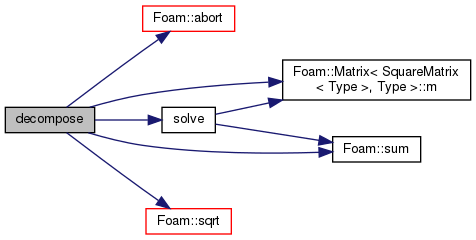## ◆ solve() [1/2]

 void solve ( Field< Type > & x, const Field< Type > & source ) const

Solve the linear system with the given source.

and returning the solution in the Field argument x. This function may be called with the same field for x and source.

Definition at line 93 of file LLTMatrix.C.

References Matrix< SquareMatrix< Type >, Type >::m(), and Foam::sum().

Referenced by LLTMatrix< Type >::decompose().

Here is the call graph for this function: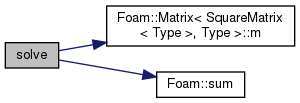Here is the caller graph for this function: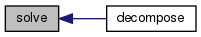## ◆ solve() [2/2]

 Foam::tmp< Foam::Field< Type > > solve ( const Field< Type > & source ) const

Solve the linear system with the given source.

returning the solution

Definition at line 136 of file LLTMatrix.C.

References tmp< T >::ref(), and solve().

Here is the call graph for this function: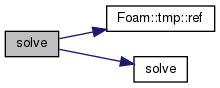The documentation for this class was generated from the following files: